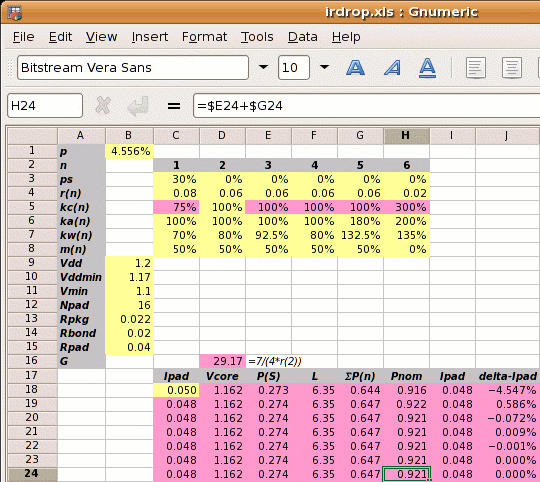We can check the result by using the strap widths and other chip specs to calculate the power that can be delivered. We use the procedure shown previously.

Step 1: Estimate Vcore using Ipad=50mA:

Vcore =
1.17×(1−2×0.050×(0.022+0.02+0.04)⁄1.2
1.162V

Step 2: Calculate the reference power supply conductance G:

G =
 7 4×r2

7 ⁄ (4 × 0.06) =  29.17 mhos

Step 3 is to set out the values of kan, kwn, kcn and mn for each metal layer, and use these to calculate the value of L.

metal layer 1 2 3 4 5 6
kan 100% 100% 100% 100% 180% 200%
power metal allocated coefficient
kwn  70%  80% 92.5%  80% 132.5% 135%
power metal used coefficient
kcn  75%¹ 100% 100% 100% 100% 300%²
conductivity coefficient
mn  50%  50%  50%  50%  50%   0%
core area blocked
¹75%=.06/.08; ²300%=.06/.02

From which

 L = kw1kc1(1-ps)(1-m1(1-ka2p)(1-ka3p))+ kw2kc2(1-m2(1-ka2p)(1-ka3p))+ kw3kc3(1-m3(1-ka2p)(1-ka3p))+ kw4kc4(1-m4(1-ka2p)(1-ka3p))+ kw5kc5(1-m5(1-ka2p)(1-ka3p))+ kw6kc6(1-m6(1-ka2p)(1-ka3p)) = ( 0.20 + 0.44 + 0.50 + 0.44 + 0.72 + 4.05 ) = 6.35

Step 4: Calculate Pnom. Pnom depends on the value of Ipad which we don't know, so we start with Ipad=50mA which gives us Vcore, then Pnom and a new value of Ipad. We use a spreadsheet to iterate to the solution. The yellow squares mark user input and the pink squares are calculated values. The first estimate for Pnom is shown below.

 m1′ = m1×(1-ka2p)(1-ka3p)
Pnom=
 { (Vcore−Vmin)×Vdd2 } ×G×(kc1×ps(1−m1′)+p×L) Vddmin
=
 { (1.162‑1.1)×1.22 } ×29.2×(.75×.3×.045+.046×6.35) 1.17
=  2.226×(0.123+0.289) = 0.916W

The iteration leads to the solution of Pnom=0.921W. This is slightly higher than the 0.9W of the example, which we expect because the power strap allocation percentage has been made slightly higher by the rounding up process.

Design Attribute Typical Value
p metal power supply allocation percentage 4.556%¹
ps fraction of metal-1 in the standard cells used for power supplies 30% (for wsclib)
r1 resistivity of metal-1 in ohms per square 0.08Ω per sq.
r2-5 resistivity of metal-2 to metal-5 in ohms per square 0.06Ω per sq.
r6 resistivity of metal-6 in ohms per square 0.02Ω per sq.
ka1-4
 user defined   ratio of metal layer n allocated to power metal-2 allocated to power
100%
ka5 180%
ka6 200%
kw1
 user defined   ratio of metal layer n used for power metal-2 allocated to power
70%
kw2,4 80%
kw3 92.5%
kw5 132.5%
kw6 135%
m1-5 percentage of metal layers 1-5 which is blocked 50%
m6 percentage of metal layer 6 which is blocked 0%
Vdd the nominal supply voltage 1.2V
Vddmin minimum supply voltage, 2.5% less than nominal 1.17V
Vmin the desired voltage at the centre of the die 1.1V